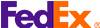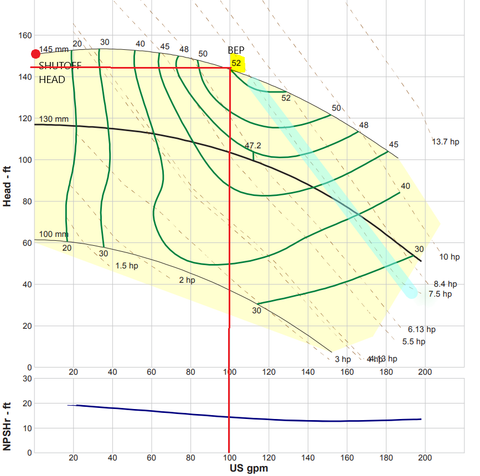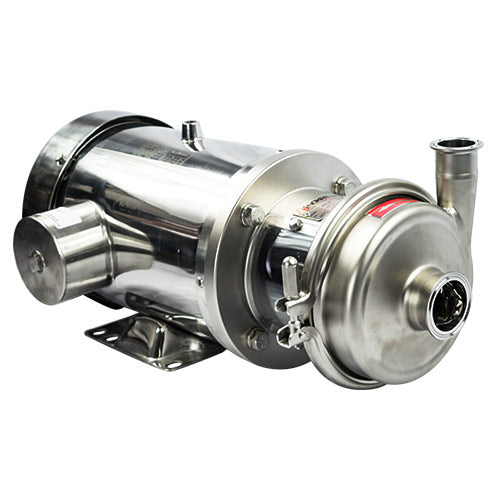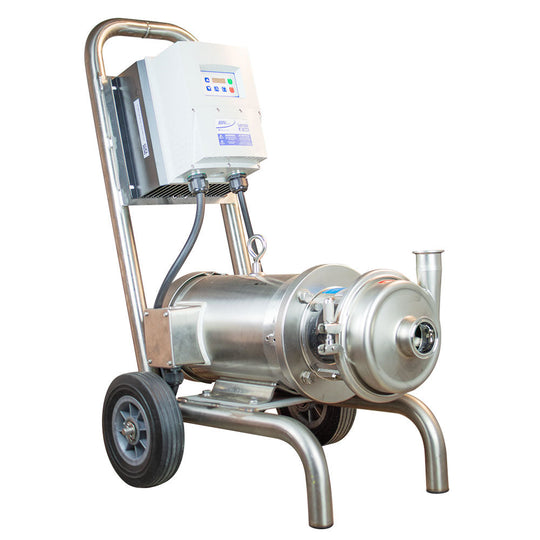We ship with FedEx across the USA and Canada

Centrifugal pumps are the most popular and widely used pump type for pumping liquids.  These pumps use a rotating impeller to move water or other liquids using centrifugal force. They  are popular in many industries for  delivering fluids from one place to another. When discussing the size of centrifugal pumps the key parameters are total head, speed and horsepower. The INOXPA Hyginox SEN-20 CENTRIFUGAL PUMP will be used as an example for these calculations.Figure 1: INOXPA HYGINOX SEN-20 CENTRIFUGAL PUMP CURVE

When determining the pump size and equipment to be installed, the total dynamic head of the water system must be considered, and the various head losses that the pump must overcome must be determined. Dynamic total head = elevation height + friction head loss + pressure head

Elevation height is the vertical distance you need to pump. This is the height difference between the pump surface level (source tank) and receiving tank.

Friction loss is the pressure loss caused by the flow of water through pipes and fittings. The diameter, length, and type of pipe material that the water flows through, from source tank  to receiving tank, is all taken into consideration. In order to determine these losses, we use the friction loss tables to determine the friction head loss due to the pipe. Friction losses can be overcome by using larger pipes.

Pressure Head is the maximum working pressure of the system based on water, converted from head pressure (psi) to feet. When calculating this you need to include the pressure drop through other equipment in the line, such as filters or heat exchangers. Use the following conversion to convert psi to feet: 1 psi = 2.31 feet altitude.

The total head produced by the pump is proportional to the speed and diameter of the impeller, so the speed or diameter of the impeller can be increased to obtain a higher head if required.

Net Positive Suction Head required (NPSHr curve) provides information about the suction power of the pump at different flow rates. The X axis is still measured in units of flow, but the Y axis is now measured in feet of NPSHr. Each point on the curve defines the NPSHr  that the pump needs in order to avoid cavitation problems that can negatively affect overall performance and damage the pump.

## The Performance Curve

The performance curve is a graph of the relationship between total head and flow rate for a given impeller diameter and speed (Figure 1). The left-most side of this graph denotes  zero flow. The output head at this point corresponds to the shutoff head of the pump, in the example above this will be about 150ft. From this point, the output head decreases until it reaches the maximum flow at zero head.  This is the performance range for this particular centrifugal pump at this speed.

The efficiency of the pump varies within its working range. This information is important for calculating motor power. The BEP (Best Efficiency Point) is the highest efficiency point of the pump. Using the INOXPA Hyginox SEN-20 CENTRIFUGAL PUMP as an example, all points on the right or left side of 52% are less efficient have small axial and radial forces on the impeller. When the operating point  is moved further away from BEP, the axial and radial forces will increase. These forces can result in vibrations and noises in extreme cases depending on the speed and design of the pump.

Performance horsepower curves represent the performance required to operate the pump within a specific range. The selected motor can reach all points on the power curve to the left of the selected power. Using the INOXPA Hyginox SEN-20 CENTRIFUGAL PUMP as an example, in order to achieve the best efficiency point the pump will require the 145mm (5.71”) impeller diameter with a 7.5 HP motor at a flow rate of 100GPM. The power can be calculated based on the total head, flow and efficiency during operation.

### Formulas:

Centrifugal Pump Flow Rate Calculation

Q = V/T

Derivation of the Flow Rate Formula

Q = refers to the liquid flow rate measured as GPM

V = Volume of fluid in Gallons

T = refers to time in minutes

hp = PQ/1714

#### Derivation of the Pump Power  Formula

Hp = fluid Power

P = Pressure in psi

Q = Flow rate in GPM

#### Pump Efficiency

Volumetric Efficiency = Actual Flow Rate Output (GPM) / Theoretical Flow Rate Output (GPM) x 100Hyginox SEN-20 Check it out Hyginox SEN-20 Assembly Check it out
Sale

Unavailable

Sold Out